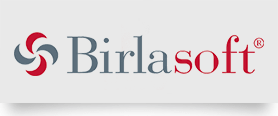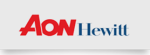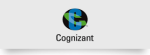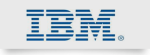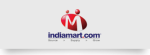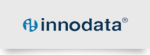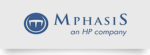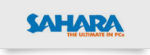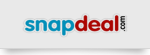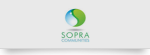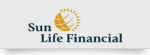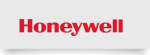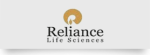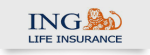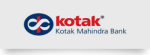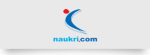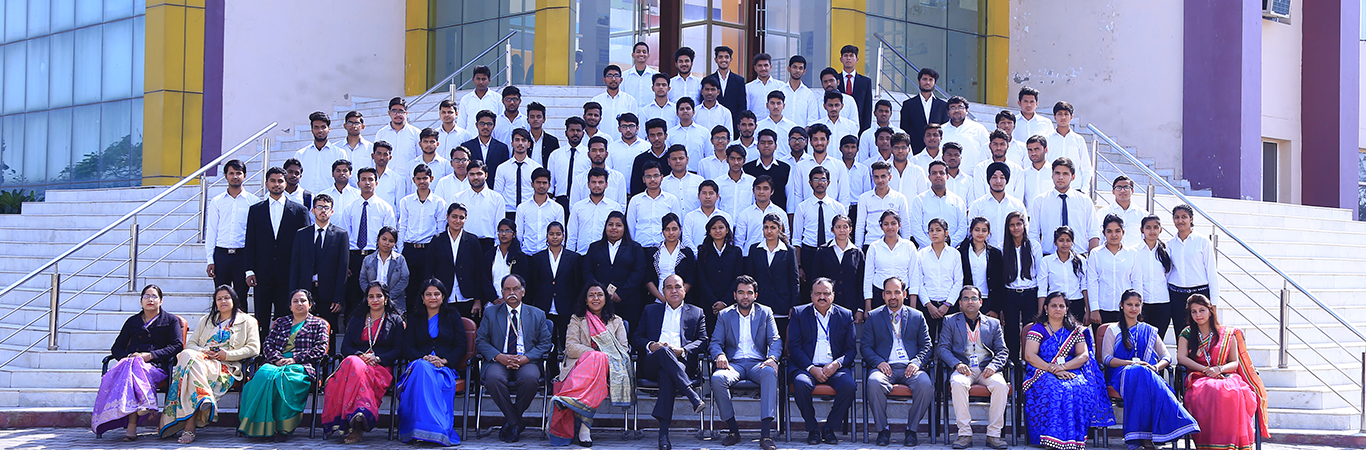## LABORATORIES

FCPC lab
This is the basic programming lab which is common to all branches. Students learn programming in C language in Turbo C++ compiler. Students also learned to design algorithm and flow charts and designing of programs from the algorithms.
Physics Lab
This is the basic science lab which is common to all branches. Students learn the basic principles of fundamental physics like laser technology, sound, electrical, optics, fiber optics, superconductivity, photovoltaics, Hall Effect and electronics, etc.
Chemistry Lab
In the chemistry lab, the B.Tech students perform experiments related to polymer science, titrations dealing with determination of hardness as well as alkalinity in water, use of instruments such as digital balance, spectrophotometer, conductivity cell and Pensky-Martin apparatus, etc.
BME Lab
In the basics of mechanical engineering lab students perform experiments related to boliers,strokes engines(2 stroke and 4 stroke),cranes machines,power transmissions elements(clutches,gears ) etc.
E.G.D lab
In the engineering graphics and drawing lab,we introduce basic designs and graphics of the various objects along with its projections to the students.
Language Lab
A language laboratory is a very recent innovation. Here listening and speaking practice is given to the individual learner. In this laboratory we help the students to learn pronunciation, self correction and self examination. We give here planned practice, controlled conversation etc.
ET Lab
This lab introduces the concept of practical implementation of electrical circuits and their application to the students. The experiments included in the curriculum are designed to demonstrate the theoretical concepts presented in the class room. Some extra value added experiments also included in this laboratory.

This lab provides the hand-on practical sessions on the theorem like Norton, Thevenin, Superposition, Maximum power transfer theorem etc. students also get trained in handling machines like Transformer, AC machine and DC Machine. All experiments are well documented with clear circuit diagram, theory and procedure involved.